# GRE Geometry PuzzleKIC Education

Updated on Apr 29, 2021

Exams Know-how | 4 min read
~ 1949 views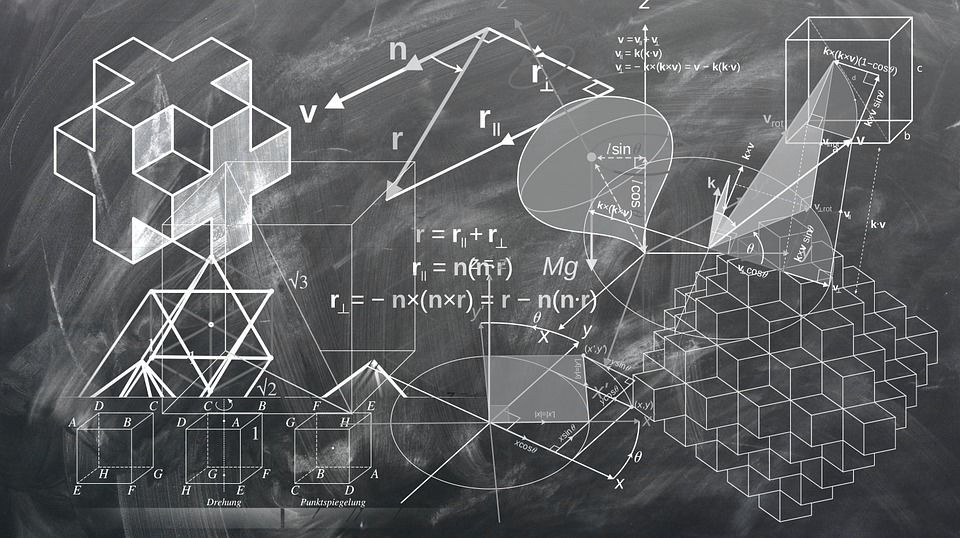Geometry is one of the challenging topics in mathematics. Your test is about concept, application and strategy. Instead of solving a conventional GRE problem, I will solve a geometry puzzle in today’s article.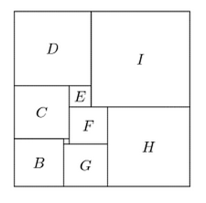The figure above has 9 squares labeled from A to I. (A is the smallest square and it is not labeled). The length of the side of square A is 1. You have to find the area of the above figure.

Now, before you look at the solution, think about the problem for a couple of minutes. You only need to know that

1. The area of a rectangle is length times breadth and
2. All the sides of a square are equal.

That is the concept. I can assume that everybody knows the two concepts that have been mentioned above. The only catch here is the way in which you apply it.
So let us start with the solution. At present, you know the length of the side of just one square, i.e. the smallest square, A.
Let us assume, that the side of square G is ‘x’ units.
Can we connect square G, with any other square using square A?
If you observe, square B has a side which is the sum of the sides of A and G.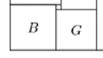A part of the figure has been redrawn so that you may be able to visualise better. The little sqaure between B and G is A.
So, we can say that the side of B is ‘x+1’ units.
Can we connect square B to another square? Again observe that, the side of C is the side of B plus the side of A.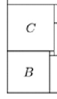So, the side of square B would be ‘x+2’ units.
Similarly, the side of square F, would be 1 less than the side of square G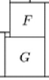We can list the sides of the squares we know at this point

A = 1
B = x + 1
C = x + 2
G = x – 1

May be, you can choose to pause here and attempt solving the question further. Give it a try and if you are not able to, see the remainder of the solution.
Let us observe another portion of the figure,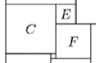The sum of the sides of squares E and F would be equal to the sum of the sides of A and C

E + x – 1 = x + 2 +1
E = 4.

So, the side of E is 4.
Side of D is, C + E = x + 2 + 4
= x + 6 (the figure below helps you visualise)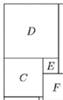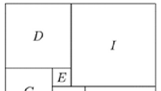From the above portion, you can conclude that the side of square I would be the sum of D and E.

That comes out to be

x + 6 + 4 = x + 10

Similarly, the side of H would be x + x – 1 = 2x - 1
We shall now list the sides of all the squares,

A = 1
B = x + 1
C = x + 2
D = x + 6
E = 4
F = x - 1
G = x
H = 2x - 1
I = x + 10

But you need the area, the answer is a number, you need to get rid of the variable x in order to get a numerical answer.
The side of I can also be obtained as H + F – E

2x – 1 + x -1 – 4 = 3x – 6

This can be equated to the side we have,

x + 10 = 3x – 6
2x = 16
x = 8

The problem becomes straight-forward now. The length of the figure (rectangle) would be B + G + H

= x + 1 + x + 2x – 1 = 4x = 32

The breadth of the rectangle would be B + C + D = x + 1 + x + 2 + x + 6 = 3x + 9 = 33.
So the area of the rectangle is 32 x 33 = 1056.
A question like this is unlikely to appear on your test, but it forces you to think in a different way. The concepts involved are very simple, but you need to know how to apply them in the real world.

#### Similar Articles:GRE FAQs: Questions About The GRE
Exams Know-how | 3 min readHow To Prepare For TOEFL
Exams Know-how | 2 min readBlogs

POPULAR COURSES

Masters

Bachelors

TOP UNIVERSITIES

By Country

By Course

YOCKET TOOLS & SERVICES